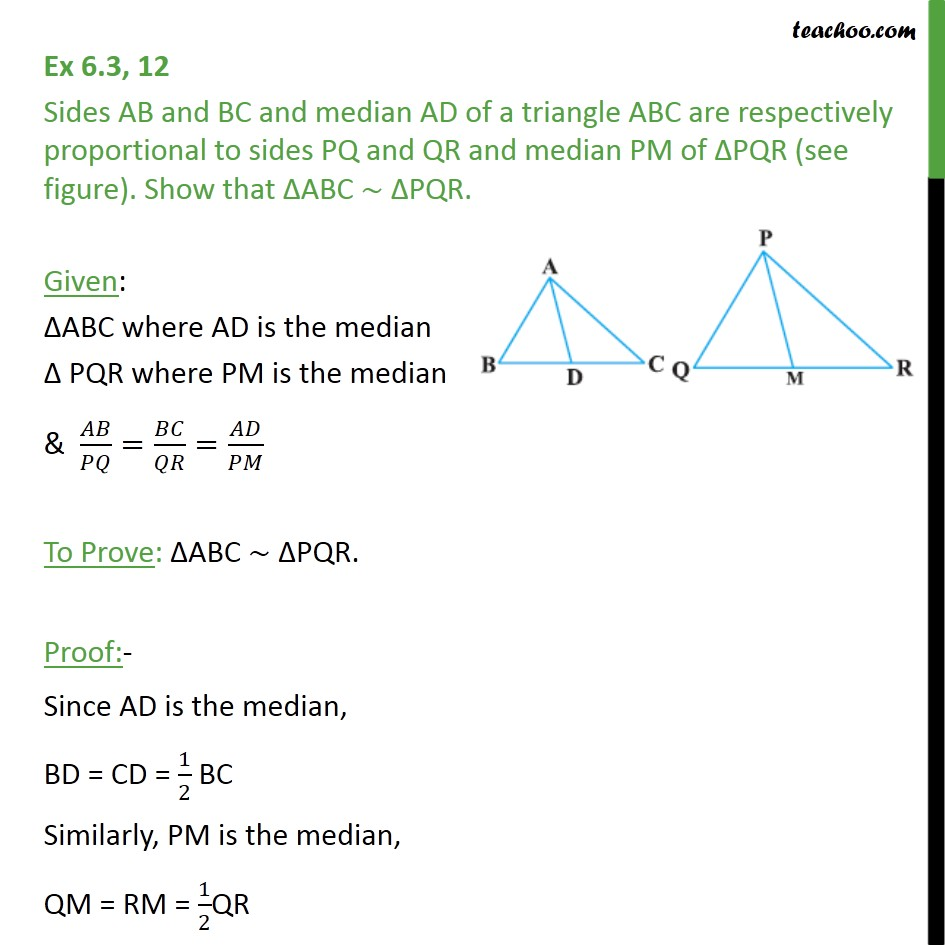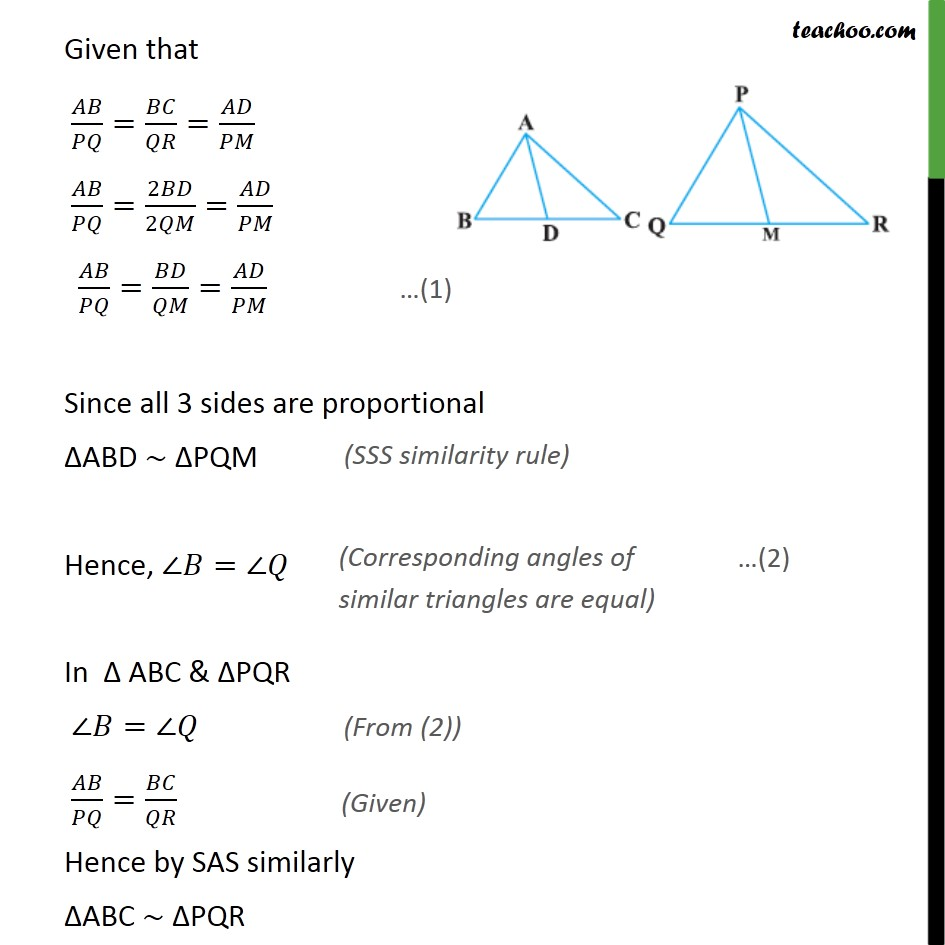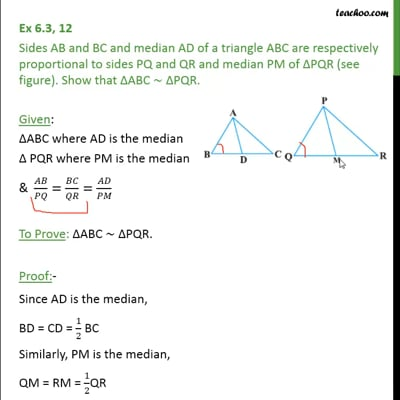SAS Similarity

Chapter 6 Class 10 Triangles
Concept wiseThis video is only available for Teachoo black users

Introducing your new favourite teacher - Teachoo Black, at only ₹83 per month

### Transcript

Ex 6.3, 12 Sides AB and BC and median AD of a triangle ABC are respectively proportional to sides PQ and QR and median PM of PQR (see figure). Show that ABC PQR. Given: ABC where AD is the median PQR where PM is the median & / = / = / To Prove: ABC PQR. Proof:- Since AD is the median, BD = CD = 1/2 BC Similarly, PM is the median, QM = RM = 1/2QR Given that / = / = / / =2 /2 = / / = / = / Since all 3 sides are proportional ABD PQM Hence, = In ABC & PQR = / = / Hence by SAS similarly ABC PQR